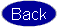Burn-In Optimization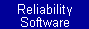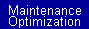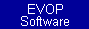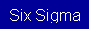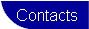Overview

Burn in is designed to expose latent defects, preventing sub-standard units from being delivered to the customer. Thus, burn-in is only useful if the population has latent defects. A population with latent defects is characterized by a decreasing hazard rate. A population with a decreasing hazard rate will have a Weibull shape parameter less than one.  Click Here for information on determining the parameters of the Weibull distribution.

Optimization Model

The burn-in optimization model uses the following variables.

t = burn-in duration
w = warranty period
c1 = burn-in cost per unit time
c2 = cost of a failure in the field during the warranty period
c3 = cost of a failure during the burn-in period
b = the shape parameter of the Weibull distribution that represents the time to fail for the population
q = the scale parameter of the Weibull distribution that represents the time to fail for the population

When fitting the Weibull distribution to the population failure data, the data must be collected on units that have not been burned-in. If the units have been burned-in, then the fitted distribution models the field performance of burned-in units, not the population prior to burn-in.

The goal of burn-in is to minimize the total system costs. The system cost is the total of

• the cost of conducting burn in burn (tc1)
• the cost of units failed during burn-in ([the probability of failing during burn-in]c3)
• cost of units failing during the warranty period in ([the probability of failing during the warranty period]c2)

The system cost is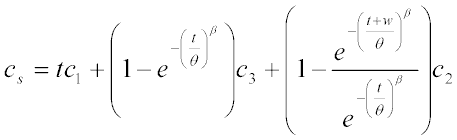The optimum burn-in time is found by minimizing equation 1 in terms of t. This can be done graphically or by using numerical methods.

Example

Historical data from a population with no burn-in yields a Weibull time to fail distribution with a shape parameter of 0.8 and a scale parameter of 5000 days. The warranty period is 700 days, and the average cost of a warranty claim is \$500. The cost of burn-in is \$0.07 per hour per item, and the cost of a failure during burn-in is \$3.00. Determine the optimum burn-in period.

Solution

The system cost is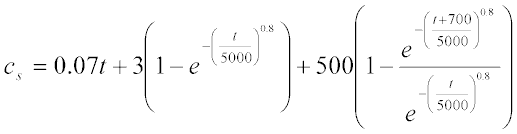The figure below shows the system cost as a function of the burn-in duration. The optimum burn-in duration is approximately 47 hours.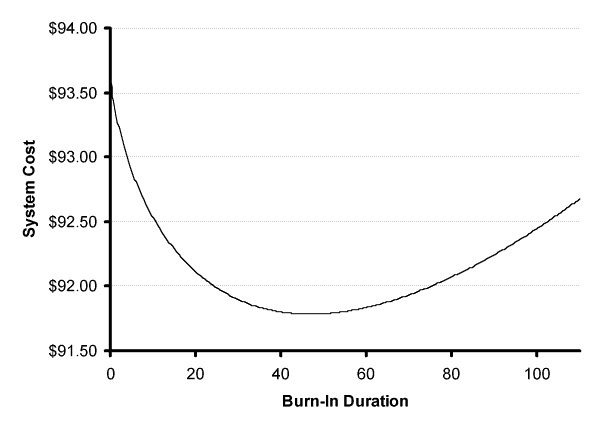The equation above can also be optimized using numerical methods. Numerical methods are tedious, but Microsoft Excel includes a tool called "Solver" that makes the process simple.

Set up a spreadsheet similar to the one shown below.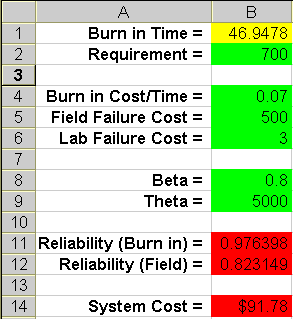The objective is to minimize cell B14 by changing cell B1. Click the Tools menu in Excel and then select Solver. If Solver does not appear on the menu select Add-Ins and check the box next to Solver Add-In. After selecting the Solver tool from the menu, complete the following steps:

1. The target cell is the cell that contains the system cost. Set the target cell to B14.
2. The system cost is to be minimized. Select the Min radio below the target cell box.
3. The target cell is minimized by changing the burn-in duration which is in cell B1. Set the By Changing Cells box to cell B1.
4. The burn-in duration must be greater than zero. This restriction is guaranteed by adding a constraint. Click the Add button to the right of the Subject to Constraints frame. Another window will appear. Input B1 for the cell reference, zero for the constraint, and >= for the operator.

These settings are shown in the figure below.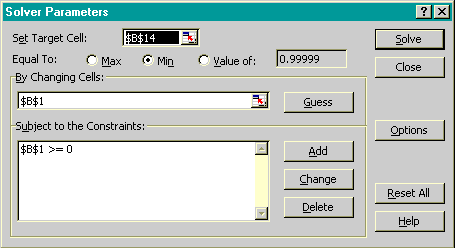Click the Solve button to get the optimum burn-in duration. Solver is dependent on the initial guess at the optimum solution and often fails to find a solution. If this occurs, change the burn-in duration and try again. There will always be an optimum solution as long as the shape parameter is less than 1 and the field failure cost is greater than the burn-in failure cost.